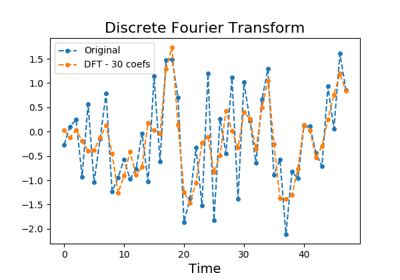# `pyts.approximation`.DiscreteFourierTransform¶

class `pyts.approximation.``DiscreteFourierTransform`(n_coefs=None, drop_sum=False, anova=False, norm_mean=False, norm_std=False)[source]

Discrete Fourier Transform.

Parameters: n_coefs : None, int or float (default = None) The number of Fourier coefficients to keep. If None, all the Fourier coeeficients are kept. If an integer, the `n_coefs` most significant Fourier coefficients are returned if `anova=True`, otherwise the first `n_coefs` Fourier coefficients are returned. If a float, it represents a percentage of the size of each time series and must be between 0 and 1. The number of coefficients will be computed as `ceil(n_coefs * (n_timestamps - 1))` if `drop_sum=True` and `ceil(n_coefs * n_timestamps)` if `drop_sum=False`. drop_sum : bool (default = False) If True, the first Fourier coefficient (i.e. the sum of the subseries) is dropped. Otherwise, it is kept. anova : bool (default = False) If True, the Fourier coefficient selection is done via a one-way ANOVA test. If False, the first Fourier coefficients are selected. norm_mean : bool (default = False) If True, center each time series before scaling. norm_std : bool (default = False) If True, scale each time series to unit variance.

References

 [R15c669394ac6-1] P. Schäfer, and M. Högqvist, “SFA: A Symbolic Fourier Approximation and Index for Similarity Search in High Dimensional Datasets”, International Conference on Extending Database Technology, 15, 516-527 (2012).

Examples

```>>> from pyts.approximation import DiscreteFourierTransform
>>> X, _, _, _ = load_gunpoint(return_X_y=True)
>>> transformer = DiscreteFourierTransform(n_coefs=4)
>>> X_new = transformer.fit_transform(X)
>>> X_new.shape
(50, 4)
```
Attributes: support_ : array, shape = (n_coefs,) Indices of the kept Fourier coefficients.

Methods

 `__init__`(self[, n_coefs, drop_sum, anova, …]) Initialize self. `fit`(self, X[, y]) Learn indices of the Fourier coefficients to keep. `fit_transform`(self, X[, y]) Learn and return the Fourier coeeficients to keep. `get_params`(self[, deep]) Get parameters for this estimator. `set_params`(self, \*\*params) Set the parameters of this estimator. `transform`(self, X) Return the selected Fourier coefficients for each sample.
`__init__`(self, n_coefs=None, drop_sum=False, anova=False, norm_mean=False, norm_std=False)[source]

Initialize self. See help(type(self)) for accurate signature.

`fit`(self, X, y=None)[source]

Learn indices of the Fourier coefficients to keep.

Parameters: X : array-like, shape = (n_samples, n_timestamps) Training vector. y : None or array-like, shape = (n_samples,) (default = None) Class labels for each data sample. Only used if `anova=True`. self : object
`fit_transform`(self, X, y=None)[source]

Learn and return the Fourier coeeficients to keep.

Parameters: X : array-like, shape = (n_samples, n_timestamps) Training vector, where n_samples in the number of samples and n_features is the number of features. y : None or array-like, shape = (n_samples,) (default = None) Class labels for each data sample. X_new : array, shape (n_samples, n_coefs) The selected Fourier coefficients for each sample.
`get_params`(self, deep=True)

Get parameters for this estimator.

Parameters: deep : bool, default=True If True, will return the parameters for this estimator and contained subobjects that are estimators. params : mapping of string to any Parameter names mapped to their values.
`set_params`(self, **params)

Set the parameters of this estimator.

The method works on simple estimators as well as on nested objects (such as pipelines). The latter have parameters of the form `<component>__<parameter>` so that it’s possible to update each component of a nested object.

Parameters: **params : dict Estimator parameters. self : object Estimator instance.
`transform`(self, X)[source]

Return the selected Fourier coefficients for each sample.

Parameters: X : array-like, shape (n_samples, n_timestamps) Input data. X_new : array, shape (n_samples, n_coefs) The selected Fourier coefficients for each sample.

## Examples using `pyts.approximation.DiscreteFourierTransform`¶Discrete Fourier Transform